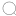# 衣柜的面积怎么算的？投影面积和展开面积你弄明白了吗？4张顶底板=4×1.7×0.6=4.08㎡

3张立板=3×2.82×0.6=5.076㎡

3张搁板=3×0.5×0.5=0.75㎡

1张背板=1.7×2.75=4.675㎡